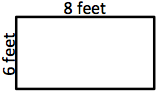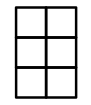Area & Perimeter
4 minutes ago
mssalas
Save
Edit
Host a game
Live GameLive
Homework
Solo Practice
Practice16 QuestionsShow answers
• Question 1
300 seconds
Q. How do you find the area of a rectangle?
Length + Length + Length + Length
Length + Width
Length x Width
Width x Width
• Question 2
300 secondsQ. Find the area.
16 cm2
30 cm2
32 cm2
60 cm2
• Question 3
300 seconds
Q. The distance around an object is called the
area
perimeter
• Question 4
900 seconds
Q. If I wanted to place a fence around my backyard to keep my dog from running away, I would be looking at?
Perimeter
Area
• Question 5
900 seconds
Q. Ashley wants to use ribbon to put a border around her picture. The picture is 6 inches long and 4 inches wide. How much border will she need?
24 inches
20 inches
10 inches
• Question 6
900 secondsQ. What is the perimeter of the rectangle?
14 sq feet
28 feet
48 feet
48 sq feet
• Question 7
900 secondsQ. What is the perimeter?
6 units
10 units
7 units
100 sq units
• Question 8
30 secondsQ. What is the area of this object?
48 square units
45 square units
14 square units
10 square units
• Question 9
180 seconds
Q. A lawn had an area of 35 square feet. If it was 7 feet width, how long was it?
84 ft.
245 ft.
5 ft.
14 ft.
• Question 10
120 seconds
Q. Adam was painting a wall in his room. The wall was 4 feet wide and 4 feet tall. What is the area of the wall he has to paint?
16ft
8ft
12ft
4ft
• Question 11
180 seconds
Q. A rectangle flower bed had a total area of 35 square yards. If it was 5 yards wide, how long was it?
175 yards
7 yards
15 yards
30 yards
• Question 12
180 seconds
Q. At the playground, the new sandbox was 10 meters wide and had an area of 60 square meters. How long is the sandbox?
600 meters
60 meters
6000 meters
6 meters
• Question 13
120 seconds
Q. A video game map was 3 meters wide and 5 meters long, what is the area of the map?
30 meters
26 meters
15 meters
8 meters
• Question 14
120 seconds
Q. A book had a length of 5 inches and a width of 8 inches. What is the perimeter of the book?
26 inches
40 inches
36 inches
32 inches
• Question 15
120 seconds
Q. A piece of plywood was cut so its length was 6 feet by 5 feet. What is the perimeter of the wood?
30 feet
22 feet
11 feet
30 feet
• Question 16
120 seconds
Q. Sarah had a sheet of paper that was 3 inches long and 7 inches wide. What is the perimeter of the paper?